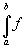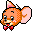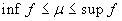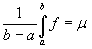www.batmath.it

### The mean value theorem

Suppose that the function f(x) is non negative and continuous in the closed interval [a,b]. Then the Riemann integralexists and represents the area bounded by the curve y=f(x) and the lines y=0 (x-axis), x=a and x=b. Consider a rectangle with bases on the x-axis between a and b, and with the same area as the integral. Then its heightmust represent the mean value of the function. Of course, the restriction that the function is non-negative (and also that of being continuous) is not necessary and can be removed: they are only useful to simplify geometrical interpretation.

Example The average value of the function f(x)=x2 on the interval [0,2] is 4/3 (remember we have proved that the integral is 8/3).

This property is generalized to integrable functions by the followingMean value theorem

Suppose that f is integrable in the closed interval [a,b]. Then there exists a number μ, with, such that. The number μ is called the mean value of f in the interval [a,b].If the function f is continuous, there exists a number c in the interval [a,b] such that f(c)=μ. Unfortunately this theorem gives no hint in order to find the number μ, otherwise it would be conclusive as far Riemann integrals are concerned.From a geometrical point of view this means that every trapezoid is equivalent to a rectangle: as any rectangle can be transformed in a square (by rule and compass), it's usual to say that every trapezoid can be squared.

first published on january 07 2003 - last updated on september 01 2003